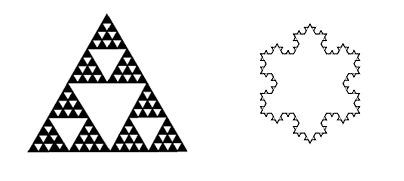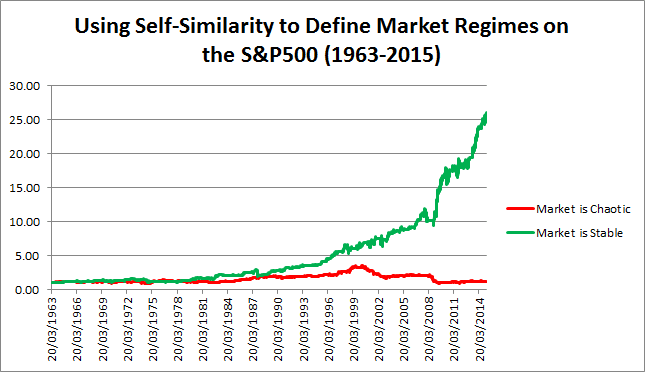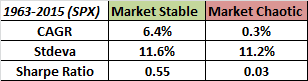September 13

# Using a Self-Similarity Metric with Intraday Data to Define Market Regimes

By EasyLanguage Mastery Contributor

September 13, 2021

EasyLanguage, Market RegimeThe images above are the famous Sierpinski Triangle and the Koch Snowflake. These objects are “self-similar” and this means that examination at finer levels of resolution will reveal the same shape. Both are examples of “fractal” geometry, and are characteristic of many phenomena in the natural world such as mountains, crystals, and gases. Self-similar objects are associated with simplicity, redundancy and hence robustness.

Self-dissimilar objects are associated with complexity and chaos. Several mathematicians (including Mandelbrot) have observed that markets are clearly non-gaussian or non-normal. Markets exhibit “fat-tails” and have a distribution that shares more in common with a Levy distribution than the normal distribution which is used frequently in quantitative finance. But the market does not have a constant distribution - at times the market behavior is fairly normal in character while at other times the market is wild and unpredictable.

The question is how we can effectively determine which regime the market is in so that we can apply the appropriate trading strategies to mitigate risk.

The essence of self-similarity and complexity is to compare the whole to its component parts. For example, let's take a square that is divided into four separate squares of equal size. The area of the larger square is equivalent to the sum of the areas of each of its component squares. The same of course is true of a one-dimensional line which is equivalent to the sum of its parts. One of the methods of identifying self-similarity in the stock market is to look at the range or the difference between the highs and the lows.

We would expect that in a perfectly self-similar market the longer range would be equivalent to the sum of the ranges measured over a smaller interval. The more chaotic the market is, the greater the difference will be between these two measures. Such market conditions would be characterized by a large ratio between the sum of smaller ranges versus the longer measure of range. Essentially this relationship is called fractal dimension and is a measure of complexity. There are many different ways to measure this including using the Hurst exponent, but the problem I have always found in my own humble research is that the suggested thresholds defined by specific absolute values do not seem to reflect the information consistent with theory.

I have often found that relative measures tend to be more robust and consistent - much the same way that the magnitude of past returns has less predictive value than the relative rank of past returns. Relative measures tend to be more stationary than absolute values. To compute this measure of self-similarity I use the intraday range (high minus low) versus a longer range window. Here is how it is calculated:

1. Find the high minus the low for each day going back 10 days.
2. Take the sum of these values (sum of the pieces).
3. Find the 10-day range by taking the 10-day maximum (including the highs) and subtracting out the 10-day minimum (whole range).
4. Divide the sum of the pieces by the whole range - this is a basic measure of fractal dimension/complexity.
5. Take the 60-day average of the 10-day series of the complexity values - this is the quarterly “chaos/stability” metric.
6. Use either the 252-day normsdist of the z-score or the percentile ranking of the chaos/stability metric.
7. Values above .5 indicate that the market is in a “chaos” regime and is much less predictable and non-stationary, values below .5 indicate that the market is stable and much more predictable.

When the market is “stable” it is easier to apply effective quantitative trading systems. When the market is in “chaos” mode, it is not necessarily volatile but rather it is too complex to use for standard measurement and calibration of basic linear prediction. Let’s look at how this measure performs over a long time period using the S&P500 as a test set. The high and low values are generally the same until about 1963 which is when we will begin this test. Here is how the market performed in both regimes over the last 50+ years:The market performs quite poorly in “chaos” conditions and seems to make all of its long-term returns in the “stable” regime. Note however that the volatility is not materially different between both regimes - this means that we are capturing something different than just high and low volatility market conditions.

Furthermore, the correlation between the chaos indicator signals and for example the basic trend signal of a 200-day moving average is -.116. This means that we are capturing something different than just the market trend as well.

The indicator is meant to be used to define regimes rather than as a trading signal to go long or short, but clearly there are some interesting attributes worthy of further exploration and refinement.

• By David Varadi of CSSA

EasyLanguage Mastery Contributor

## Capture The Big Moves! (Updated 2022)

•Nik says:

Hi,
code with Multicharts not work, as a compilation error

• Hey Nick, I’m not familiar with Multicharts so maybe someone else will have an answer. What’s the error?

•John says:

Thank you much, Jeff, for your attempt to code it for Trade Station.

•John says:

Yes, red and green numbers/values showing.

•Nik says:

06.04.15 19:04:23
—— Build started: ——
Study: “CSSA Regime” (Indicator)
—— Compiled with error(s): ——
unrecognized token: !

line 42, column 14

Plot1( pRank, !( “CSSA Regime” ) ) ;
Plot2(.5, !( “Threshold” ) ) ;

• Here is something to try. Do you get this error when you attempt to compile from the ELD, or from the text file? If you get it after importing the ELD, what happens if you create an indicator by using the text file?

•Nik says:

is the same

•Paul Mayer says:

It was easy to fix the text code to work with Multicharts. Just edit the plot commands to remove the ! and the extra ( ) for each plot. Then the indicator shows up fine. However, when I converted that same code into a LE LX signal (buy when pRank crosses above .5 and close when pRank crosses below .5) and applied it to SPX daily, it gives very different results than the author; in fact it loses money.

I like the concept of knowing when markets are behaving “normally”, but this particular code seems to need more work.

• The indicator code was just updated today. If you have an earlier version you will want to grab the latest copy.

•Rick says:

Good article. Trying to implement indicator in excel. What value do you get for S&P 500 after close of Tuesday, April 7? Just a check. Q: Are parameters fixed or should vary from market to market?

•Sam says:

This is an indicator of inverse momentum. It has nothing to do with randomness. When > 0.5, market has less momentum and when < 0.5 market has more momentum.

• The PercentRank formula doesn’t look correct to me. The way I read it you should be ranking the AvgMetric against its past 252 values not against RawMetric.

pRank = PercentRank( AvgMetric, RawMetric, 252 );

Use this:
pRank = PercentRank( AvgMetric, AvgMetric, 252 );

• Agreed. I’ve updated the code. Thanks!

•Bob says:

I wish the author could participate in the discussion. There are some questions here. Is this fitted to S&P 500? What about oil of gold? How is this different from a momentum indicator?

• As a follow up…TradeStation’s PercentRank function is dreadfully slow. I found a much faster function (_PercentRankFast) on the internet here: http://dvindicators.cssanalytics.com/community/?vasthtmlaction=viewtopic&t=47.0

Here is the text file for the _PercentRankFast function (you’ll find it is MUCH faster than the TS built-in one):

inputs:
ValueToRank(numericsimple),
DataSet(numericseries),
Length(numericsimple);
variables:
R(0),
X(0);

R = 0;
for X = 1 to Length begin
if DataSet[X-1] <= ValueToRank then
R = R + 1;
end;

_PercentRankFast = R / ( Length – 1 ) ;

•Mark says:

Is the graph simply the performance of SPY if you were to go long when the market is “stable” (green) or “chaotic” (red)?

Even if that is the case, isn’t it a leap to say quantitative trading systems in general work better when the market is stable as opposed to chaotic?

• Not sure if that’s what the author did. But you’re right that you need to test this regime filter with your particular market and strategy.

•Michele says:

Hi Mr. Jeff Swanson,
first of all many thanks for sharing your code and for your very useful article. Am i allowed to code it in my own R package naming and linking you?
Thanks.
Michele Stasulli

•aaron says:

Has any one done tested this regime filter on a very basic mean reversion strategy (like 2 period RSI) and a very basic trend following one (like 20 period donchian channel breakout) ??? would be curious to see how the regime indicator adds to those types of strategies.